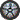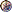### Prof. Paulo Franca - Spring 2000

Introduction

Syllabus

Guidelines for labs

Lab sections

Take quiz & check grades

### Hashing TablesMotivation: If key can be used as index to array, search is O(1).Keys as index may not be practical in several situations.

### Hash tables:

Try to achieve efficiency of arrays.

##### Hashing Functions:

map a key into an index for the array. If array has N entries, key should be mapped to an integer between 0 and N-1 (or 1 and N). Assume the key is an integer or may be treated as an integer.Division Method: Using an array of size N, divide the key by N and take the remainder as an index. Some values of N are better than others. Typically, use a prime N.Multiplication Method: Divide the key by a number A ( 0
##### Collision:

Some keys will map to same index. How will you handle collisions?Linked list: Instead of array of records, use an array of linked lists of records.collision resolution by chainingOpen addressing: Use vacant positions of the array for the colliding records

#### Properties:number of elements stored: nnumber of indexes in the array: mload factor: (alfa= n / m)simple uniform hashing: assume each element is equaly likely to hash into any of the m slots

#### Theorem 12.1:

Hash table with collisions handled by chaining, unsuccessful search takes Teta(1+alfa) on the average.

#### Theorem 12.2:

Hash table with collisions handled by chaining, successful search takes Teta(1+alfa) on the average.